# NCERT Solutions For Class 9 Maths Chapter 1 – Number System

Download NCERT Solutions For Class 9 Maths Chapter 1 Number System – Exercise 1.1, 1.2, 1.3, 1.4, 1.5, 1.6

## NCERT Solutions For Class 9 Maths Chapter 1 – Exercise 1.1

1. Is zero a rational number? Can you write it in the form p/q, where p and q are integers
and q ≠ 0?

Solution 1

Yes , 0 is a rational number because it can be written in the form p/q = 0/1, p= 0 and q = 1 i.e. where p, q are integers and q ≠ 0 .

2. Find six rational numbers between 3 and 4.

Solution 2

To find six rational numbers between 3 and 4 follow the steps below:

Step (I) Write 3 and 4 as rational numbers with denominator 6+1 = 7

Thus, 3 = 3/1

= (3 x 7)/(1 x 7)

= 21/7

Similarly, 4 = 4/1

= (4 x 7)/(1 x 7)

= 28/7

Step (II) Write the numbers between the given numbers as-

22/7, 23/7, 24/7, 25/7, 26/7, 27/7

Since all have same denominator , we can easily observe that all of these numbers are in between 21/7 and 28/7 i.e. 3 and 4 respectively.

3. Find five rational numbers between 3/5 and 4/5.

Solution 3

To find five rational numbers between 3/5 and 4/5 follow the steps below:

Step (I) Write 3/5 and 4/5 as rational numbers with denominator 5 x (5+1) = 30
Thus 3/5 = (3 x 6)/(5 x 6) = 18/30

Similarly, 4/5 = (4 x 6)/(5 x 6) = 24/30

Step (II) Write the numbers between the given numbers as-

19/30, 20/30, 21/30, 22/30, 23/30

or , 19/30, 2/3, 7/30, 11/15, 23/30 in their simplest forms respectively.

4. State whether the following statements are true or false. Give reasons for your answers.
(i)Every natural number is a whole number.
(ii) Every integer is a whole number.
(iii) Every rational number is a whole number.

Solution 4

(i) True , because Whole number is the collection of Natural numbers including Zero.
(ii) False , -1 or any negative integer is not a whole number.
(iii) False , because not all the numbers which can be expressed in the form p/q, where p and q are integers and q≠0 , are necessarily Whole numbers. Example 3/5 is a rational number but not a whole number.

## NCERT Solutions For Class 9 Maths Chapter 1 – Exercise 1.2

1.State whether the following statements are true or false. Justify your answers.
(i) Every irrational number is a real number.
(ii) Every point on the number line is of the form √m , where m is a natural number.
(iii) Every real number is an irrational number.

Solution 1

(i) True , because real number is the collection of rational and irrational numbers.
(ii) False , for example 1/2 is not of √m form with m as a natural number and also since square root of a natural number is considered to be positive so we can not represent negative numbers in this form.
(iii) False , because every rational number is a real number but not irrational number.

2. Are the square roots of all positive integers irrational? If not, give an example of the square root of a number that is a rational number.

Solution 2

No, For example consider the integer 9
√9 = 3 which is a rational number

3. Show how √5 can be represented on the number line.

Solution: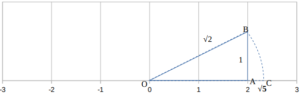Follow the steps below to represent √5 on the number line as show in the figure above:

Step (I) : Consider a right angled triangle OAB on the number line as shown in figure with
OA = 2 units
AB = 1 unit.
Then by using Pythagoras theorem we can easily find
OB = √(2² + 1²) = √5
Step (II) : Using a compass , draw an arc with centre O and radius OB intersecting the number line at C. Then C corresponds to √5 on the number line.

4. Classroom activity

## NCERT Solutions For Class 9 Maths Chapter 1 – Exercise 1.3

1. Write the following in decimal form and say what kind of decimal expansion each has :

(i) 36/100

(ii) 1/11

(iii) 4 1⁄8

(iv) 3/13

(v) 2/11

(vi) 329/400

Solution:

(i) 36/100 = 0.36 , it is terminating decimal

We can check by performing long division as follows: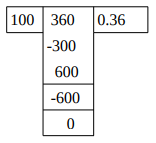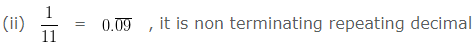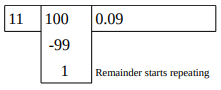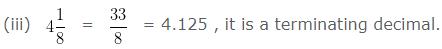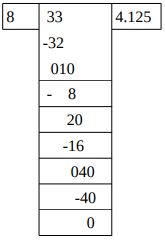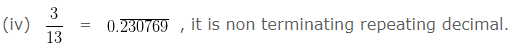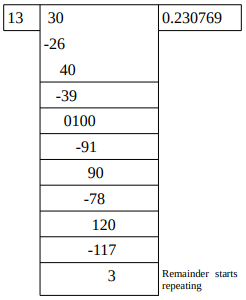(v) 2/11 = 2 x 1/11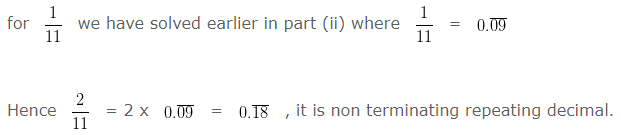(vi) 329/400 = 0.8225 , it is a terminating decimal.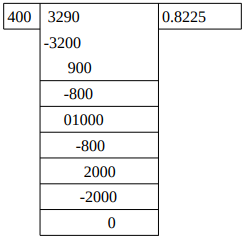(2). You know that 1/7 = 0.142857 . Can you predict what the decimal expansions of 2/7, 3/7, 4/7, 5/7, 6/7 are, without actually doing the long division? If so, how?

[Hint : Study the remainders while finding the value of 1/7 carefully.]

Solution:

While performing long division for 1/7.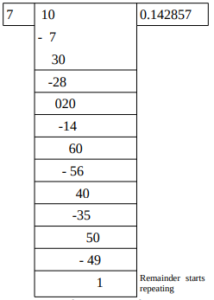We can easily observe that each one from the numerator of the given fractions appear in the remainder of the above operation which has to be carried out further by dividing them by 7 . And so just by using this observation we can predict the required decimal expansions as follows: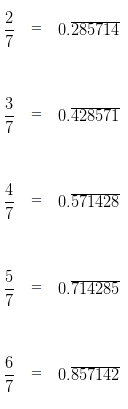(3). Express the following in the form p/q, where p and q are integers and q ≠ 0.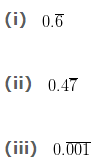Solution:

(i) Follow the steps below :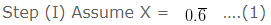Step (II) Since there is only one repeating block , multiply X by 10
We get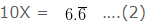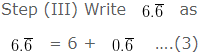Step (IV) Using equation (1) and (2) , equation (3) can be written as
10X = 6 + X ….(4)
Now,
Solving for X from equation (4) , we get
9X  =  6
Therefore,
X = 6/9 = 2/3
Hence we obtain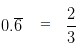(ii) Follow the steps below :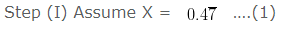Step (II) Since there is only one repeating block , multiply X by 10
We get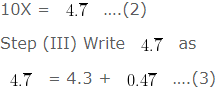Step (IV) Using equation (1) and (2) , equation (3) can be written as
10X = 4.3 + X  ….(4)
Now,
Solving for X from equation (4) , we get
9X  =  4.3
Therefore,
X = (4.3/9) = (43/90)
Hence we obtain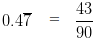(iii) Follow the steps below :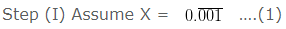Step (II) Since there are three repeating block , multiply X by 1000
We get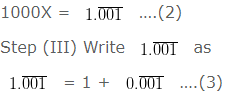Step (IV) Using equation (1) and (2) , equation (3) can be written as
1000X = 1 + X  ….(4)
Now,
Solving for X from equation (4) , we get
999X = 1
Therefore,
X = (1/999)
Hence we obtain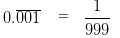(4). Express 0.99999…. in the form p/q . Are you surprised by your answer? With your teacher and classmates discuss why the answer makes sense.

Solution:

(i) Follow the steps below to write 0.9999…. in the form p/q: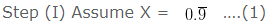Step (II) Since there is only one repeating block , multiply X by 10
We get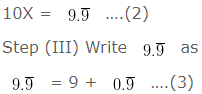Step (IV) Using equation (1) and (2) , equation (3) can be written as
10X = 9 + X ….(4)
Now,
Solving for X from equation (4) , we get
9X = 9
Therefore,
X = 9/9 = 1
Hence we obtain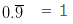(5). What can the maximum number of digits be in the repeating block of digits in the decimal expansion of 1/17 ? Perform the division to check your answer.

Solution:
The maximum number of digits in the repeating block must be less than the denominator i.e. 17. So the mximum number of digits in the repeating block in this case is 16.
Perform the long division for 1/17 as follows: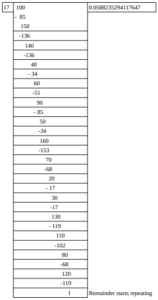Thus we obtained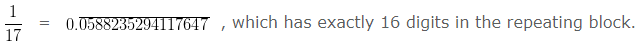(6). Look at several examples of rational numbers in the form p/q (q ≠ 0), where p and q are integers with no common factors other than 1 and having terminating decimal representations (expansions). Can you guess what property q must satisfy?

Solution:
We can easily observe that in such rational numbers q , apart from 1 and itself ; is either divided by 2 or 5 or both.
So we can say that the prime factorization of q must have only powers of 2 or 5 or both apart from 1 and the number itself.

(7). Write three numbers whose decimal expansions are non-terminating non-recurring.

Solution:

There are numerous number of such examples , three of them are as follows:
0.1011011101111011111……..
0.0100100010000100000……..
0.2122122212222122222……..

(8). Find three different irrational numbers between the rational numbers 5/7 and 9/11.
Solution:

We can calculate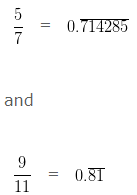Out of infinite possibilities three are as follows :
0.72722722272222……
0.7277277727777……..
0.80800800080000……

(9). Classify the following numbers as rational or irrational :
(i) √23
(ii) √225
(iii) 0.3796
(iv) 7.478478…
(v) 1.101001000100001…

Solution:

(i) √23 : Irrational
(ii) √225 = 15 : Rational
(iii)0.3796 : Rational
(iv)7.478478… : Rational
(v)1.101001000100001… : Irrational.

## NCERT Solutions For Class 9 Maths Chapter 1 – Exercise 1.4

NCERT Solutions For Class 9 Maths Chapter 1 Exercise 1.4 – Number System

1. Visualise 3.765 on the number line, using successive magnification.

Solution:

Follow the steps below to visualise 3.765 on the number line :
Step (I) We know that 3.765 lies between 3 and 4 . So , first we will divide this portion of number line into 10 parts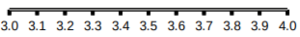Step (II) We know that 3.765 lies between 3.7 and 3.8 . So now , we will divide this portion of number line into 10 parts.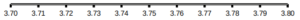Step (III) We know that 3.765 lies between 3.76 and 3.77 . So now , we will divide this portion of number line into 10 parts.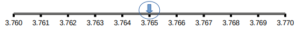Thus we obtained 3.765 on the number line, using successive magnification.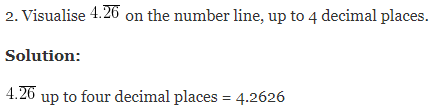Follow the steps below to visualise 4.2626 on the number line :
Step (I) We know that 4.2626 lies between 4 and 5 . So , first we will divide this portion of number line into 10 parts.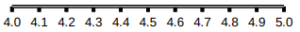Step (II) We know that 4.2626 lies between 4.2 and 4.3 . So now , we will divide this portion of number line into 10 parts.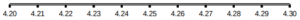Step (III) We know that 4.2626 lies between 4.26 and 4.27 . So now , we will divide this portion of number line into 10 parts.Step (IV) We know that 4.2626 lies between 4.262 and 4.263 . So now , we will divide this portion of number line into 10 parts.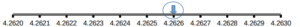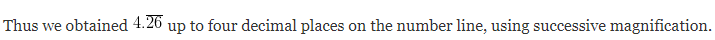## NCERT Solutions For Class 9 Maths Chapter 1 – Exercise 1.5

1. Classify the following numbers as rational or irrational:
(i) 2 – √5
(ii) ( 3 + √23 ) – √23
(iii) (2√7/7√7)
(iv) 1/√2
(v) 2π

Solution:

(i) 2 – √5 : It is irrational.
(ii) ( 3 + √23 ) – √23 = 3 + √23 – √23 = 3 : It is rational.
(iii) (2√7/7√7) = 2/7 : It is rational
(iv) 1/√2 = : It is irrational.
(v) 2π : It is irrational.

(2). Simplify each of the following expressions:
(i)( 3 + √3 )( 2 + √2 )
(ii)( 3 + √3 )( 3 – √3 )
(iii)(√5+√2)²
(iv)( √5 – √2 )( √5 + √2 )

Solution:
(i) ( 3 + √3 )( 2 + √2 )
( 3 + √3 )( 2 + √2 )
= ( 3 + √3 )2 +( 3 + √3 )√2
= 6 + 2√3 + 3√2 + √6

(ii)( 3 + √3 )( 3 – √3 )
Using the identity
( a + b )( a – b) = a2 – b2
We get
( 3 + √3 )( 3 – √3 ) = 3² – √32 = 9 – 3 = 6

(iii) (√5+√2)²
Using the identity
(a + b)² = a2 + 2ab + b2
We get
(√5+√2)² = 5 + 2 √10 + 2 = 7 + 2 √10

(iv)( √5 – √2 )( √5 + √2 )
Using the identity
( a – b )( a + b) = a2 – b2
We get
( √5 – √2 )( √5 + √2 ) = 5 – 2 = 3

(3) Recall, π is defined as the ratio of the circumference (say c) of a circle to its diameter (say d). That is, π = c/d. This seems to contradict the fact that π is irrational. How will you resolve this contradiction?

Solution:

As we measure c and d using any physical device we do not get the exact value manually , instead we get an approximate rational value and this is why this contradiction arises.

4. Represent √9.3 on the number line.

Solution: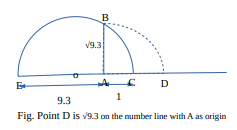Follow the steps below to locate √9.3 on the number line as shown above:
Step (I) Draw a line segment of length 9.3 units EA and extend it to C by 1 unit .
Step (II) Now with O as mid point of EC draw a semicircle with O as center and OC as radius.
Step (III) Draw a line perpendicular to EC at A and intersecting the semicircle at B.
Step (IV) Taking A as center and AB as radius draw an arc from B to intersect the extended line EAC at D.

If we assign A as origin i.e. 0
Then D represents √9.3 on the number line as shown in the figure.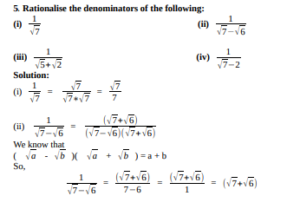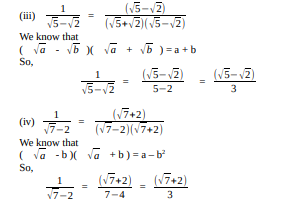## NCERT Solutions For Class 9 Maths Chapter 1 – Exercise 1.6

(1). Find :

(i)641/2
(ii) 321/5
(iii)1251/3

Solution:

(i)641/2 = (82)1/2 = 8

(ii) 321/5  = (25)1/5 = 2

(iii)1251/3= (53)1/3 = 5

(2). Find :

(i)93/2
(ii)322/5
(iii)163/4
(iv) 125-1/3

Solution:

(i)93/2  = 27

(ii)322/5   = 4

(iii)163/4 = 8

(iv) 125-1/3 = 1/5

(3). Simplify :

(i)22/3.21/5
(ii)(1/33)7
(iii)(111/2)/(111/4)
(iv)71/2.81/2

Solution:

(i)22/3.21/5

= 2{(2/3)+(1/5)}

=2{(10 + 3)/15}

= 213/15

(ii)(1/33)7

=1/321

= 3-21

(iii)(111/2)/(111/4)

= 11{(1/2) – (1/4)}

=  11{(2-1)/4}

=  111/4

(iv)71/2.81/2

=(7×8)1/2

= (56)1/2

Download NCERT Solutions For Class 9 Maths Chapter 1 Number System – Exercise 1.1, 1.2, 1.3, 1.4, 1.5, 1.6